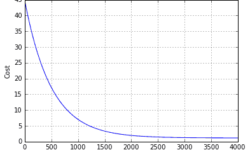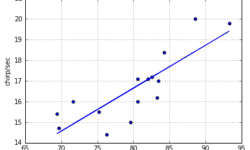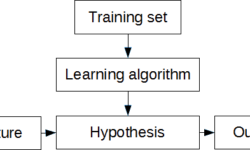## Linear regression with multiple featuresSingle feature linear regression is simple. All you need is to find a function that fits training data best. It is also easy to plot data and learning curves. But in reality, regression analysis is based on multiple features. So in most cases, we cannot imagine the multidimensional space where data could be plotted. We need to rely on the methods we use. You must feel comfortable with linear algebra, where matrices and vectors are used. If previously we had one feature (temperature), now we need to introduce more of them. So we need to expand hypothesis to accept more features. From now and later on, instead of output y, we are going to use h(x) notation: As you can see, with more variables (features), we also end up with more parameters θ that has to be learned. Before we move let’s find relevant data that we could use for building machine learning algorithm. The data set Again we are going to use data set college cengage. This time we select health data set with several variables. The data (X1, X2, X3, X4, X5) are by city. X1 = death rate per 1000 residents X2 = doctor availability per 100,000…

## Linear regression – learning algorithm with PythonIn this post, we will demystify the learning algorithm of linear regression. We will analyze the simplest univariate case with single feature X wherein the previous example was temperature and output was cricket chirps/sec. Let’s use the same data with crickets to build learning algorithm and see if it produces a similar hypothesis as in excel. As you may already know from this example, we need to find linear equation parameters θ0 and θ1, to fit line most optimally on the given data set: y = θ0 + θ1 x x here is a feature (temperature), and y – output value (chirps/sec). So how are we going to find parameters θ0 and θ1? The whole point of the learning algorithm is doing this iteratively. We need to find optimal θ0, and θ1 parameter values, so that approximation line error from the plotted training set is minimal. By doing successive corrections to randomly selected parameters we can find an optimal solution. From statistics, you probably know the Least Mean Square (LMS) algorithm. It uses gradient-based method of steepest descent.

## Simplest machine learning algorithm – linear regression with excelSome may say that linear regression is a more statistical problem. And this is true at some level. But when the problem is solved from a machine learning perspective, things gets more accessible, especially when moving towards more complex problems. First of all, let’s understand few essential terms. We can start with regression. When speaking of linear regression we try to find a best-fitting line through given points. In other words, we need to find an optimal linear equation to fit given data points. This is a supervised learning problem when we have set of data pairs that can be plotted on x-y axis. I understand theory is a boring thing, even for me, so let’s move to practical examples and learn by solving some problems. In order to work with some examples we need sample data. There are many data sources available on the internet. For instance, a great source is college Cengage, that have several sets with data pairs meant for linear regression problems. For our example, we are going to use Cricket Chirps Vs. Temperature data where each data point consists of chirps/sec and temperature in degrees Fahrenheit. You can send data in three formats: excel, mtp and…